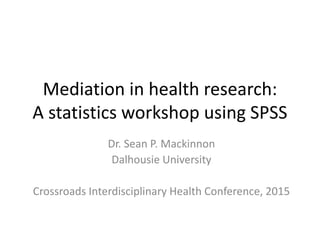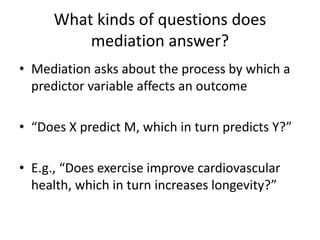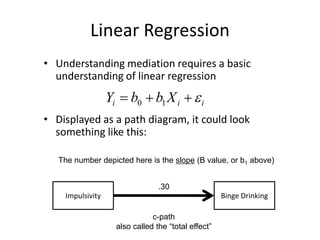Successfully reported this slideshow.

# Introduction to Mediation using SPSS

93

Share×
1 of 29
1 of 29

# Introduction to Mediation using SPSS

93

Share

An introduction to mediation analysis using SPSS software (specifically, Andrew Hayes' PROCESS macro). This was a workshop I gave at the Crossroads 2015 conference at Dalhousie University, March 27, 2015.

An introduction to mediation analysis using SPSS software (specifically, Andrew Hayes' PROCESS macro). This was a workshop I gave at the Crossroads 2015 conference at Dalhousie University, March 27, 2015.

## More Related Content

### Related Books

Free with a 14 day trial from Scribd

See all

### Related Audiobooks

Free with a 14 day trial from Scribd

See all

### Introduction to Mediation using SPSS

1. 1. Mediation in health research: A statistics workshop using SPSS Dr. Sean P. Mackinnon Dalhousie University Crossroads Interdisciplinary Health Conference, 2015
2. 2. What kinds of questions does mediation answer? • Mediation asks about the process by which a predictor variable affects an outcome • “Does X predict M, which in turn predicts Y?” • E.g., “Does exercise improve cardiovascular health, which in turn increases longevity?”
3. 3. Linear Regression • Understanding mediation requires a basic understanding of linear regression • Displayed as a path diagram, it could look something like this: Impulsivity Binge Drinking .30 The number depicted here is the slope (B value, or b1 above) c-path also called the “total effect” iii XbbY  10
4. 4. Mediation • Mediation builds on this basic linear regression model by adding a third variable (i.e., the “mediator”) • In mediation, the third variable is thought to come in between X & Y. So, X leads to the mediator, which in turn leads to Y. Impulsivity Binge Drinking Enhancement Motives
5. 5. Mediation • The idea is, the c-path (the direct effect) should get smaller with the addition of a mediator. • So, we want to know if the c-path – c’-path is “statistically significant.” Impulsivity Binge Drinking Enhancement Motives c’-path Also called the “direct effect”
6. 6. Mediation • To test this, you first need to get the slope of two other relationships: a and b paths Impulsivity Binge Drinking Enhancement Motives c’-path Get the slope of this relationship a-path Get the slope of this relationship while also controlling for enhancement motives b-path
7. 7. Mediation • Mathematicians have shown that – (a-path * b-path) = c-path – c’ path – (But only when X and M are continuous) • Thus, if a*b (“the indirect effect”) is statistically significant, mediation has occurred Impulsivity Binge Drinking Enhancement Motives c’-path a-path b-path Preacher & Hayes (2008)
8. 8. Significance of Indirect Effect • Lots of ways to test the significance of a*b – Test of Joint Significance – Sobel Test – Bootstrapped Confidence Intervals • Of these methods, bootstrapping is currently the most preferred • But … Hayes & Scharkow (2013) have shown that the different methods agree > 90% of the time…
9. 9. Joint Significance Test (Baron & Kenny, 1986) • If the a-path AND the b-path are both significant, conclude that a*b is also significant. • This is a liberal test (i.e., high Type I error) and is usually used as a supplement to other methods. Impulsivity Binge Drinking Enhancement Motives .05 .25* .28* c’ path a-path b-path
10. 10. Sobel Test (Sobel, 1982) • An alternative is to estimate the indirect effect and its significance using the Sobel test (Sobel. 1982). • It is a conservative test (i.e., high Type II error) • z-value = a*b/SQRT(b2*sa 2 + a2*sb 2) – a = B value (slope) for a-path – b = B value (slope) for b-path – sa = SE for a-path – sa = SE for b-path • Online Calculator for Sobel Test: – http://quantpsy.org/sobel/sobel.htm – Also available in the PROCESS macro discussed later
11. 11. Bootstrapping • The sobel test is inaccurate because it relies on an assumption of a normal sampling distrbution: – However, the sampling distribution distribution of a*b is non-normal except in very large samples… • Bootstrapping is a computer intensive, robust analysis technique that can be applied to non-normal data. • Virtually any analysis can be bootstrapped, but we’re going to apply it to testing the significance of the indirect effect (a*b).
12. 12. What is a “Re-Sample?” In SPSS, Each row is a “person” who has an ID, and lots of values on measures A “re-sample” randomly samples participants from the sample, with replacement Re-sample 1 ID1 ID3 ID4 ID2 Re-sample 2 ID1 ID1 ID3 ID2 Re-sample 3 ID4 ID4 ID2 ID2 Note that people can be duplicated in the resamples using this method
13. 13. What is bootstrapping? The idea of the sampling distribution of the sample mean x-bar: take very many samples, collect the x-values from each, and look at the distribution of these values From Hesterberg et al. (2003)
14. 14. What is bootstrapping? From Hesterberg et al. (2003) The theory shortcut: if we know that the population values follow a normal distribution, theory tells us that the sampling distribution of x-bar is also normal. This is known as the central limit theorem
15. 15. What is bootstrapping? From Hesterberg et al. (2003) The bootstrap idea: when theory fails and we can afford only one sample, that sample stands in for the population, and the distribution of x in many resamples stands in for the sampling distribution
16. 16. Bootstrapping Indirect Effects • Create 1000s of simulated datasets using re- sampling with replacement – Pretends as though your sample is the population, and you simulate other samples from that. • Run the analysis once in each of these 1000s of samples • Of those analyses, 95% of the generated statistics will fall between two numbers. If zero isn’t in that interval, p < .05!
17. 17. Effect Sizes for Mediation • There are many different ways to calculate effect sizes for mediation analysis (Preacher & Kelly, 2011) • Two simple-to-understand effect size measures are: – Percent mediation (PM) – Completely Standardized Indirect Effect (abcs)
18. 18. Percent Mediation Impulsivity Binge Drinking Enhancement Motives .12* (.05) .25* .28* c-path (c’ path) a-path b-path ab = .25 * .28 = .07 c = .12 PM = .07 / .12 = .583 Interpreted as the percent of the total effect (c) accounted for by your indirect effect (a*b).
19. 19. Note about Percent Mediation… • The direct effect (c’-path) can sometimes be larger than the total effect (c-path) – Inconsistent mediation • In these cases, take the absolute value of c’ before calculating effect size to avoid proportions greater than 1.0.
20. 20. Completely Standardized Indirect Effect • So, it’s just two steps: – 1. Calculate the standardized regression paths for the a and b paths – 2. Multiply them together to get the ES – (So, just standardize your variables before analysis and you can get a 95% CI!) • Is now a standardized version that will be similar in interpretation across measures … but it’s no longer bounded by -1 and 1 like a correlation. Which is the same as …
21. 21. Installing the PROCESS macro in SPSS • Download files from here: – process.spd – http://www.processmacro.org/download.html Once you do this, you’ll get a new analysis you can run under: Analyze  Regression  PROCESS Now every time you open SPSS, you’ll have the option to run mediation analyses!
22. 22. A Sample Model w. Output Conscientious Personality Overall Physical Health Health-Related Behaviours Uses a (fabricated) dataset you can find online here if you want to try it on your own time for practice: http://savvystatistics.com/wp- content/uploads/2015/03/crossroads.2015.data_.csv RQ: Do health related behaviours mediate the relationship between conscientious personality and overall physical health?
23. 23. How to Run in SPSS For basic mediation, use “model 4” Conscientiousness = X Physical health = Y Health-Related Behaviours = M
24. 24. Annotated Output: a, b. c’ paths Coeff = Slope; SE = standard error; t = t-statistic; p = p-value LLCI & ULCI = lower and upper levels for confidence interval a-path b-path c'-path (direct effect)
25. 25. Annotated Output: c-path c-path (total effect)
26. 26. Annotated Output: Effect Size & Significance of Indirect Effect Effect Size 1: abcs (Report the 95% CI For this) Effect Size 2: PM (Don’t use the 95% CI For this) Upper and Lower Bootstrapped 95% CI a*b or “indirect effect” Report the 95% CI for this If the CI for a*b does not include zero, then mediation has occurred!
27. 27. Reporting Mediation Analysis There was a significant indirect effect of conscientiousness on overall physical health through health-related behaviours, ab = 0.21, BCa CI [0.15, 0.26]. The mediator could account for roughly half of the total effect, PM = .44. Conscientious Personality Overall Physical Health Health-Related Behaviours0.52*** 0.39*** 0.26*** (0.47)***
28. 28. Questions / Contact Thank you for your time!  • Email: mackinnon.sean@dal.ca
29. 29. Appendix: Syntax *Make sure to run the process.sps macro first, or this won’t work! *This is an alternative to running using the GUI PROCESS vars = health bfi.c behave /y=health/x=bfi.c/m=behave/w=/z=/v=/q=/ model =4/boot=1000/center=0/hc3=1/effsize=1/ normal=1/coeffci=1/conf=95/percent=0/total=1/ covmy=0/jn=0/quantile =0/plot=0/contrast=0/ decimals=F10.4/covcoeff=0. 2015-03-24